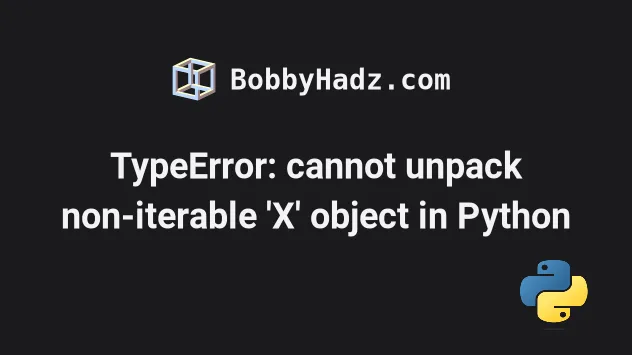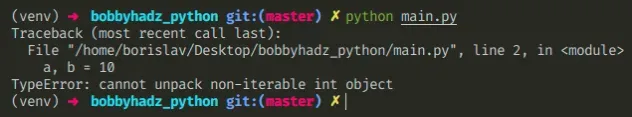# TypeError: cannot unpack non-iterable int object in PythonLast updated: Apr 20, 2022Photo from Unsplash

## TypeError: cannot unpack non-iterable int object in Python#

The Python "TypeError: cannot unpack non-iterable int object" occurs when we try to unpack an integer value. To solve the error, track down where the variable got assigned an integer and correct the assignment to an iterable, e.g. a list or a tuple of integers.Here is an example of how the error occurs.

main.py
```Copied!```# ⛔️ TypeError: cannot unpack non-iterable int object
a, b = 10
``````

We are trying to unpack an integer, but integers are not iterable.

You can use a tuple or a list of integers.

main.py
```Copied!```a, b = (10, 20)

print(a)  # 👉️ 10
print(b)  # 👉️ 20
``````

The variables need to be exactly as many as the values in the iterable.

You can use this approach if you need to initialize multiple variables to `0`.

main.py
```Copied!```a, b, c = 0, 0, 0

print(a, b, c)  # 👉️ 0, 0, 0
``````

If you are unpacking the result of calling a function, make sure to return a tuple or a list of integers from the function.

main.py
```Copied!```def get_list():
return [10, 20]

a, b = get_list()

print(a)  # 👉️ 10
print(b)  # 👉️ 20
``````

The `get_list` function returns a list of integers, so we can unpack the integers into variables.

If you need to assign individual digits of an integer to variables, convert the integer to a string.

main.py
```Copied!```a, b, c = str(123)

print(a)  # 👉️ '1'
print(b)  # 👉️ '2'
print(c)  # 👉️ '3'
``````
Unlike integers, strings are iterable. Note that the individual variables store strings, so you'll have to pass them to the `int()` class if you need to convert them back to integers.

Use an `if` statement if you need to check whether a variable doesn't store an integer before unpacking.

main.py
```Copied!```example = 10

if not isinstance(example, int):
a, b = example
print(a, b)
else:
# 👇️ this runs
print('Variable stores an integer')
``````

Alternatively, you can reassign the variable to an iterable if it stores an integer.

main.py
```Copied!```example = 10

if isinstance(example, int):
example = (0, 0)
a, b = example

print(a, b)  # 👉️ 0, 0
``````

We check if the `example` variable stores an integer and if it does, we reassign it to a tuple.

If you aren't sure what type of object a variable stores, use the `type()` class.

main.py
```Copied!```my_int = 10

print(type(my_int))  # 👉️ <class 'int'>
print(isinstance(my_int, int))  # 👉️ True

my_list = [0, 0]

print(type(my_list))  # 👉️ <class 'list'>
print(isinstance(my_list, list))  # 👉️ True
``````

The type class returns the type of an object.

The isinstance function returns `True` if the passed in object is an instance or a subclass of the passed in class.

I wrote a book in which I share everything I know about how to become a better, more efficient programmer.You can use the search field on my Home Page to filter through all of my articles.